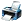# Please solve the question as soon as possible.

529 viewsposted Dec 18, 2016

+1 voteanswer Dec 20, 2016
Similar Questions

(i) Convert 14 mm to its equivalent in m.
(ii) Convert 35 kg to its equivalent in g.
(iii) Convert 57 mL to its equivalent in L.
(iv) Convert a speed of 88 m/s to its equivalent in cm/s.
(v) Convert a density of 9.45 g/L to its equivalent in g/mL.
(vi) The density of mercury metal is 1.6 g/mL. What is the mass of 3.55 mL of the metal?
(vii) The density of lead is 11.3 g/mL. What is the mass of 45 mL of the metal?
(viii) The density of table salt, NaCl, is 2.16 g/mL. what is the mass of 100.0 mL of this solid?
(ix) A particle moves through a gas at a speed of 15 km/s. How far will it move in 5.5 s?
(x) A mole of copper contains 6.02×1023 atoms. How many atoms are there in 0.525 moles?
(xi) A solution of barium nitrate contains 61.2 g per liter of solution. How many grams of barium nitrate is
contained in 2.75 L of this solution?
(xii) A sample of seawater contains 0.000245 g of sodium chloride per mL of solution. How much sodium
chloride is contained in 50.0 mL of this solution?